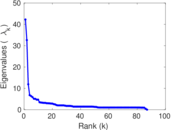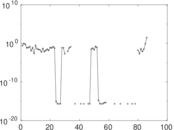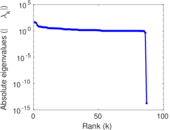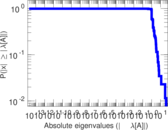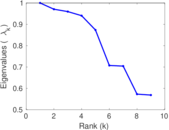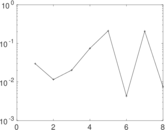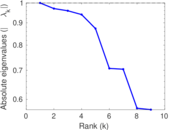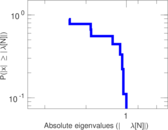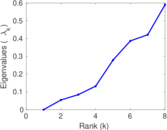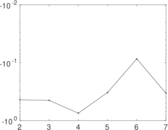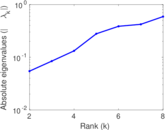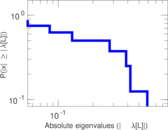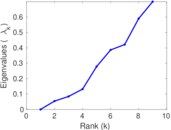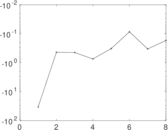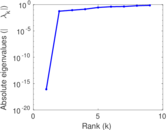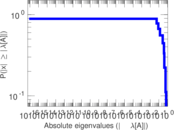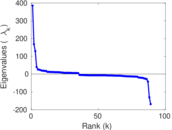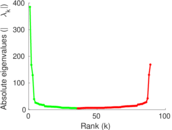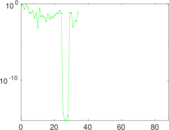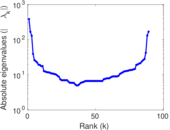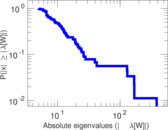# Wikibooks edits (vo)

This is the bipartite edit network of the Volapük Wikibooks. It contains users and pages from the Volapük Wikibooks, connected by edit events. Each edge represents an edit. The dataset includes the timestamp of each edit.

 Code `bvo` Internal name `edit-vowikibooks` Name Wikibooks edits (vo) Data source http://dumps.wikimedia.org/ AvailabilityDataset is available for download Consistency checkDataset passed all tests Category Authorship network Dataset timestamp 2017-10-20 Node meaning User, article Edge meaning Edit Network formatBipartite, undirected Edge typeUnweighted, multiple edges Temporal dataEdges are annotated with timestamps

## Statistics

 Size n = 394 Left size n1 = 89 Right size n2 = 305 Volume m = 499 Unique edge count m̿ = 347 Wedge count s = 6,689 Claw count z = 223,062 Cross count x = 5,991,209 Square count q = 22 4-Tour count T4 = 27,642 Maximum degree dmax = 121 Maximum left degree d1max = 121 Maximum right degree d2max = 42 Average degree d = 2.532 99 Average left degree d1 = 5.606 74 Average right degree d2 = 1.636 07 Fill p = 0.012 783 2 Average edge multiplicity m̃ = 1.438 04 Size of LCC N = 145 Diameter δ = 7 50-Percentile effective diameter δ0.5 = 1.814 40 90-Percentile effective diameter δ0.9 = 3.803 05 Median distance δM = 2 Mean distance δm = 2.757 42 Gini coefficient G = 0.602 937 Balanced inequality ratio P = 0.263 527 Left balanced inequality ratio P1 = 0.226 453 Right balanced inequality ratio P2 = 0.376 754 Relative edge distribution entropy Her = 0.875 236 Power law exponent γ = 5.479 19 Tail power law exponent γt = 2.721 00 Tail power law exponent with p γ3 = 2.721 00 p-value p = 0.478 000 Left tail power law exponent with p γ3,1 = 2.171 00 Left p-value p1 = 0.115 000 Right tail power law exponent with p γ3,2 = 3.611 00 Right p-value p2 = 0.101 000 Degree assortativity ρ = −0.262 633 Degree assortativity p-value pρ = 6.976 14 × 10−7 Spectral norm α = 42.237 4 Algebraic connectivity a = 0.054 384 9 Spectral separation |λ1[A] / λ2[A]| = 1.295 71 Controllability C = 219 Relative controllability Cr = 0.557 252

## Plots

### Fruchterman–Reingold graph drawing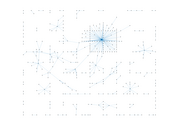### Degree distribution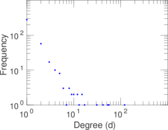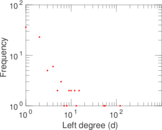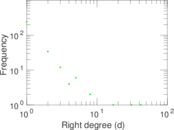### Cumulative degree distribution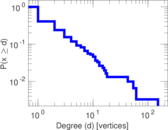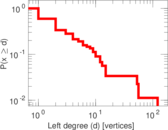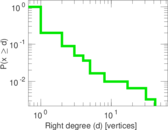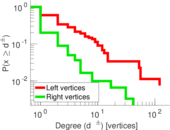### Lorenz curve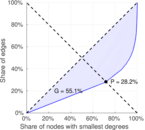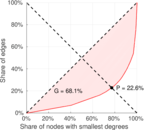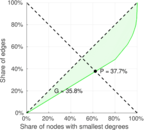### Spectral distribution of the adjacency matrix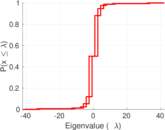### Spectral distribution of the normalized adjacency matrix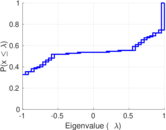### Spectral distribution of the Laplacian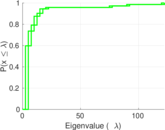### Spectral graph drawing based on the adjacency matrix### Spectral graph drawing based on the Laplacian### Spectral graph drawing based on the normalized adjacency matrix### Degree assortativity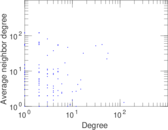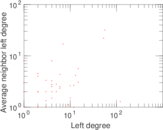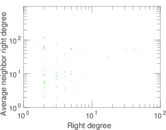### Zipf plot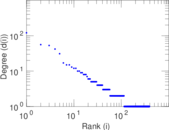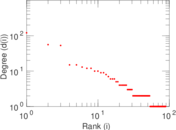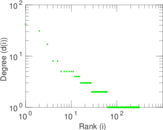### Hop distribution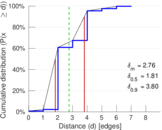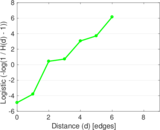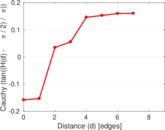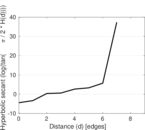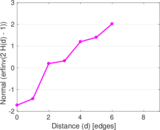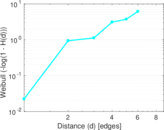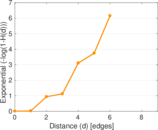### Double Laplacian graph drawing### Delaunay graph drawing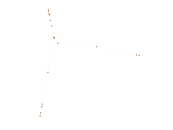### Edge weight/multiplicity distribution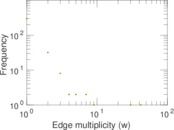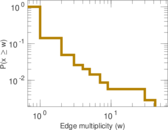### Temporal distribution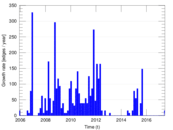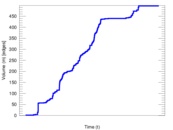### Temporal hop distribution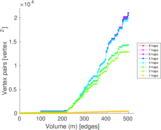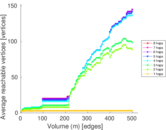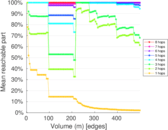### Diameter/density evolution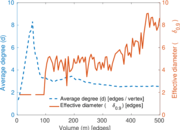### Matrix decompositions plots